Traveltime approximations for transversely isotropic media with an inhomogeneous backgroundNext: Appendix B: Expansion in Up: Alkhalifah: TI traveltimes in Previous: Bibliography

# Appendix A: Expansion inTo derive a traveltime equation in terms of perturbations in, we first establish the form for the governing equation for TI media given by the eikonal representation. The eikonal equation for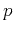-waves in TI media in 2D (for simplicity) is given by(25)

To solve equation A-1 through perturbation theory, we assume thatis small, and thus, a trial solution can be expressed as a series expansion ingiven by(26)

where,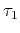and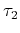are coefficients of the expansion given in units of traveltime, and, for practicality, terminated at the second power of. Inserting the trial solution, equation A-2, into equation A-1 yields a long formula, but by setting, I obtain the zeroth-order term given by(27)

which is the eikonal formula for VTI anisotropy. By equating the coefficients of the powers of the independent parameter, in succession, we end up first with the coefficients of first-power in, simplified by using equation A-3, and given by(28)

which is a first-order linear partial differential equation in. The coefficient of, with some manipulation, has the following form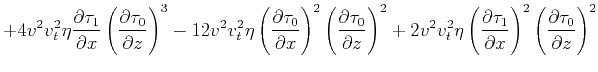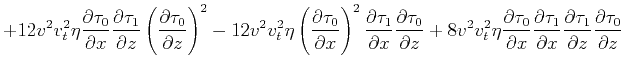(29)

which is again a first-order linear partial differential equation inwith an obviously more complicated source function given by the right-hand side. Though the equation seems complicated, many of the variables of the source function (right-hand side) can be evaluated during the evaluation of equations A-3 and A-4 in a fashion that will not add much to the cost.Traveltime approximations for transversely isotropic media with an inhomogeneous backgroundNext: Appendix B: Expansion in Up: Alkhalifah: TI traveltimes in Previous: Bibliography

2013-04-02